#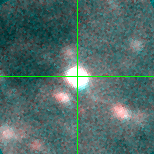Var C (J013335.14+303600.4)

## Target Notes### Classification: LBV

In a crowd but >3 magnitudes brighter than all around it.

## Plots of Brightness and Color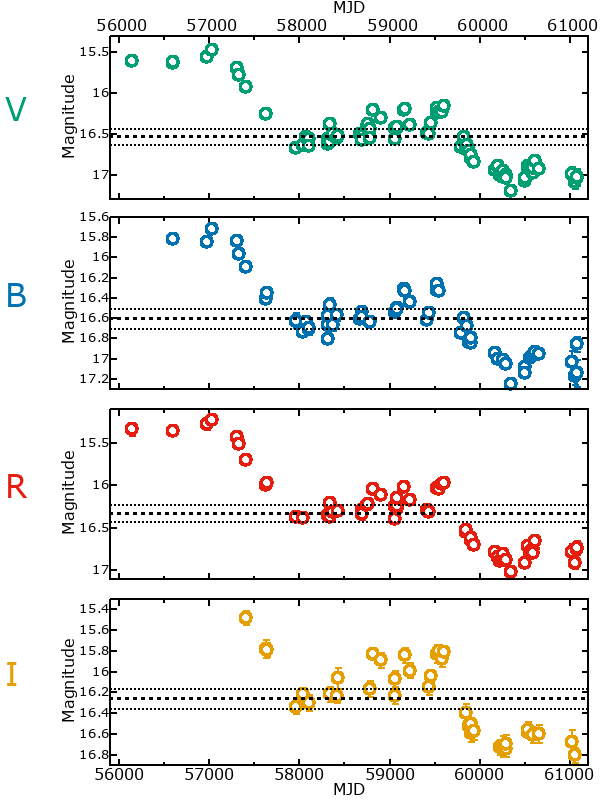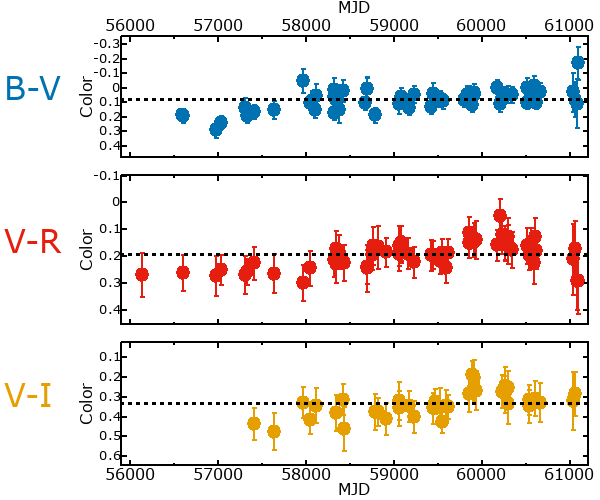## Variability

It is very likely that this star is variable.

### Correlation factors between bands

If the brightness variation is true variability then there should be a correlation between brightness changes recorded in different filter bands. The following table are the R-squared linear correlation factors calculated for the pairs of bands. The number in parentheses next to the R-squared value is the number of elements used to calculate the correlation factor. The number in square brackets next to the band is the range of magnitudes measured.

V [1.08 mag]B [1.20 mag]R [1.18 mag]
B [1.08 mag]0.98 (29)
R [1.18 mag]0.99 (26)0.98 (19)
I [0.86 mag]0.97 (15)0.91 (10)0.95 (11)

### Magnitude-Magnitude Plots

The plots below are provided to check the values in the table above and also to show any non-linear correlations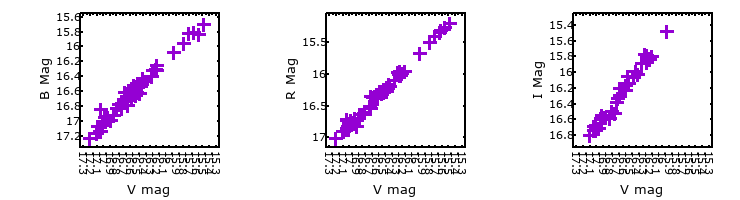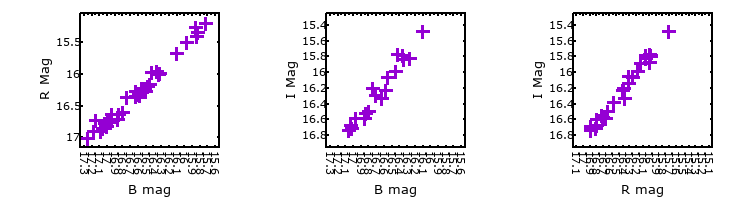### I & R Statistics

Welch & Stetson (1993, AJ, 105, 1813) present a method for varible star detection in CCD images from automated surveys. Data from two bands are compared and two statistics are calculated: R and I.

The I-statistic has an expectation value of zero for stars that are NOT variable. A larger non-zero I-value indicates a higher likelihood that the star is variable. The R statistic indicates how the color of the star changes as the variable grows brighter with R = 1.0 being no change in color.

R and I values have only been calculated if there are more than four (4) matched measurements of brightness in each band.
V
(I|R)
B
(I|R)
R
(I|R)
B
(I|R)
92.50 | 0.96
R
(I|R)
76.40 | 1.3061.25 | 1.10
I
(I|R)
16.85 | 1.5118.10 | 1.1416.49 | 1.33